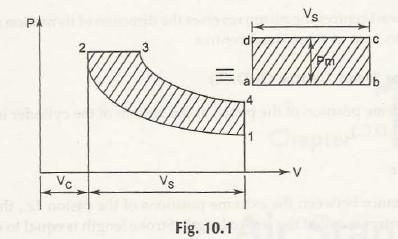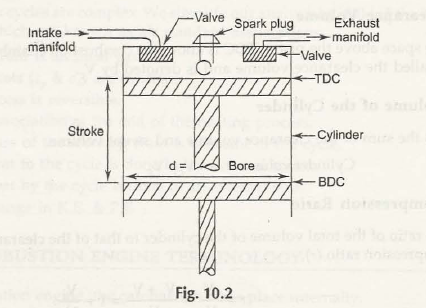Combustion takes place internally in Internal Combustion Engine

10.2.1 Bore

Bore is the inner diameter of the cylinder

The extreme piston position which is at the top of the cylinder (head side) is called as top dead centre (TDC). These are known as outer dead centre (ODC) in horizontal engines. The piston reverses the direction of its motion along with its velocity which is at the end of dead centers which is equal to zero in dead centers end.

The piston extreme position which is at the bottom of the cylinder is called as bottom dead centre (B.D.C.)

10.2.4 Stroke

The distance between the two dead centres which is also the extreme positions of the piston is known as stroke length. Stroke length is just equal to the double of the crank radius.

10.2.5 Swept Volume

The volume which is swept through piston is moving in top dead centre and bottom dead centre is known by the name as swept volume and it is denoted by V s

V s = π / 4 d2 x1

Here ,

d = cylinder bore and 1 = stroke length

10.2.6 Clearance Volume

The space which is above the piston TDC is called as combustion chamber space and the volume inside that chamber is known as clearance volume which is denoted by v c-

10.2.7 Volume of the Cylinder

Volume of the Cylinder is the total of clearance volume along with swept volume.

Cylinder volume= V=V c + V s

10.2.8 Compression Ratio

Total volume of the cylinder ratio which is that of the clearance volume is known as compression ratio (r).

r = V /V c = V c + V s / V c = 1 + V s / V c

Compression ratio changes from 5 to 10.5 in 5.1. (Spark ignition) engine and 12 to 25 for C.I.in (compression ignition) engine.

10.2.9 Mean Effective Pressure (Pro)

Mean effective pressure states the constant pressure in theoretical fieldin which the work which is done during volume changes V5 is the same work as done in net work.W net = Pm x V s

In Fig. 10.1, area 1 – 2-3-4-1 = area a – b – c-d -a.

Thus

Pm = W net / V s = Net work done per cycle / swept volume

In other words, we can say that the mean effective pressure is theoretical constant pressures which have produce similar net work during power stroke, when it is acted on piston developed in one cycle.Links of Next Mechanical Engineering Topics:-### Customer Reviews

My Homework Help
Rated 5.0 out of 5 based on 510 customer reviews at